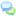# Difference between '==' (equal) and '===' (identical) comparison operators in PHP (with examples)

#### Category:

Tutorials > Web Development > PHP > Tips and Tricks

Two of the many comparison operators used by PHP are '==' (i.e. equal) and '===' (i.e. identical). The difference between the two is that '==' should be used to check if the values of the two operands are equal or not. On the other hand, '===' checks the values as well as the type of operands.

Let me explain more using some examples:

## '==' (Equal):

``` if("22" == 22) echo "YES";
else           echo "NO";
```

The code above will print "YES". The reason is that the values of the operands are equal. Whereas when we run the example code below:

## '===' (Identical):

``` if("22" === 22) echo "YES";
else            echo "NO";
```

The result we get is "NO". The reason is that although values of both operands are same their types are different, "22" (with quotes) is a string while 22 (w/o quotes) is an integer. But if we change the code above to the following:

``` if("22" === (string)22) echo "YES";
else           echo "NO";```

Then, the result will be "YES". Notice that we changed the type of right operand to a string which is the same as the left operand (i.e. string). Now, the types and values of both left and right operands are the same hence both operands are identical.

In a nutshell, whenever you want to compare the values as well as the types of operands you'll use '===' otherwise you use '=='.

Did this tutorial help a little? How about buy me a cup of coffee?Please feel free to use the comments form below if you have any questions or need more explanation on anything. I recommend thoroughy testing on a production-like test system first before moving to production.

## Comments (write a comment):

ecellent way to ans .....specially with example its totally clear Posted by: atul on Sep 17, 2013

Thank you. Its really understandable. Posted by: Lubna Mahmud on Mar 03, 2015

Thanks. Its amazing how so-o many blogs and sites make something so simple so complicated. Yet, you have managed to explain everything using simple, concise language. Posted by: Peter on May 23, 2015

simply superb explanatation... Posted by: suma on Oct 08, 2015

##leave a comment

 Notify me of follow-up comments via email.